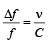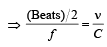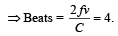Courses

# Test: Waves 1 - From Past 28 Years Questions

## 11 Questions MCQ Test Physics 28 Years Past year papers for NEET/AIPMT Class 11 | Test: Waves 1 - From Past 28 Years Questions

Description
This mock test of Test: Waves 1 - From Past 28 Years Questions for NEET helps you for every NEET entrance exam. This contains 11 Multiple Choice Questions for NEET Test: Waves 1 - From Past 28 Years Questions (mcq) to study with solutions a complete question bank. The solved questions answers in this Test: Waves 1 - From Past 28 Years Questions quiz give you a good mix of easy questions and tough questions. NEET students definitely take this Test: Waves 1 - From Past 28 Years Questions exercise for a better result in the exam. You can find other Test: Waves 1 - From Past 28 Years Questions extra questions, long questions & short questions for NEET on EduRev as well by searching above.
QUESTION: 1

### Two waves are represented by the equations y1 = a sin (ωt + kx + 0.57) m and y2 = a cos (ωt + kx) m, where x is in meter and t in sec. The phase difference between them is 

Solution:

Here, y1 = a sin (ωt + kx + 0.57)

and    y2 = a cos (ωt + kx)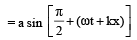Phase difference,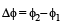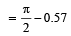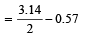= 1.57 – 0.57

QUESTION: 2

### Sound waves travel at 350 m/s through a warm air and at 3500 m/s through brass. The wavelength of a 700 Hz acoustic wave as it enters brass from warm air 

Solution:

We have, v = nλ

⇒ v ∝ λ (as n remains constant) Thus, as v in creases 1 0 times, λ also increases 10 times.

QUESTION: 3

### Two identical piano wires kept under the same tension T have a fundamental frequency of 600 Hz. The fractional increase in the tension of one of the wires which will lead to occurrence of 6 beats/s when both the wires oscillate together would be [2011M]

Solution:

For fundamental mode,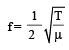Taking logarithm on both sides, we get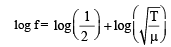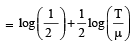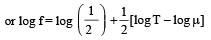Differentiating both sides, we get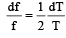(as  and μ are constants)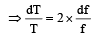Here df = 6

f = 600 Hz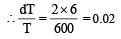QUESTION: 4

When a string is divided into three segments of length l1, l2, and l3  the fundamental frequencies of these three segments are v1, v2 and v3 respectively. The original fundamental frequency (v) of the string is 

Solution:

Fundamental frequency is given by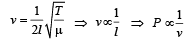Since, P divided into l1 , l2 and l3 segments

Here l = l1 + l2 + l3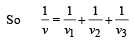QUESTION: 5

Two sources of sound placed close to each other are emitting progressive waves given by y1 = 4 sin 600πt and y2 = 5 sin 608πt. An observer located near these two sources of sound will hear :

Solution:

2πf1 = 600π
f1 = 300 ... (1)
2πf2 = 608π
f2 = 304 ...(2)
|f1 – f2| = 4 beats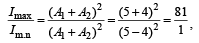where A1, A2 are amplitudes of given two sound wave.

QUESTION: 6

A train moving at a speed of 220 ms–1 towards a stationary object, emits a sound of frequency 1000 Hz. Some of the sound reaching the object gets reflected back to the train as echo. The frequency of the echo as detected by the driver of the train is : [2012M] (speed of sound in air is 330 ms–1)

Solution:

Frequency of the echo detected by the driver of the train is (According to Doppler effect in sound)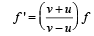where f = original frequency of source of sound f' = Apparent frequency of source because of the relative motion between source and observer.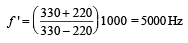QUESTION: 7

If we study the vibration of a pipe open at both ends, then which of the following statements is not true ? [NEET 2013]

Solution:

Pressure change will be minimum at both ends. In fact, pressure variation is maximum at /2 because the displacement node is pressure antinode.

QUESTION: 8

A source of unknown frequency gives 4 beats/s, when sounded with a source of known frequency 250 Hz. The second harmonic of the source of unknown frequency gives five beats per second, when sounded with a source of frequency 513 Hz. The unknown frequency is [NEET 2013]

Solution:

When sounded with a source of known frequency fundamental frequency = 250 ± 4 Hz = 254 Hz or 246 Hz
2nd harmonic if unknown frequency (suppose) 254 Hz = 2 × 254 = 508 Hz
As it gives 5 beats
∴  508 + 5 = 513 Hz
Hence, unknown frequency is 254 Hz

QUESTION: 9

A wave travelling in the +ve x-direction having displacement along y-direction as 1m, wavelen gth 2πm and frequency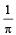Hz isrepresented by [NEET 2013]

Solution:

As Y = A sin (ωt – kx + φ)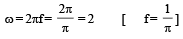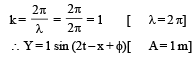QUESTION: 10

The length of the wire between two ends of a sonometer is 100 cm. What should be the positions of two bridges below the wire so that the three segments of the wire have their fundamental frequencies in the ratio of 1 : 3 : 5?              [NEET Kar. 2013]

Solution:

From formula,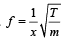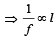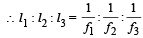= f2 f3 : f1 f3 : f1 f2
[Given: f1 : f2 : f3 = 1 : 3 : 5] = 15 : 5 : 3
Therefore the positions of two bridges below the wire are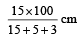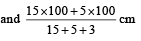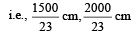QUESTION: 11

Two sources P and Q produce notes of frequency 660 Hz each. A listener moves from P to Q with a speed of 1 ms–1. If the speed of sound is 330 m/s, then the number of beats heard by the listener per second will be [NEET Kar. 2013]

Solution: